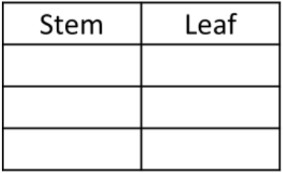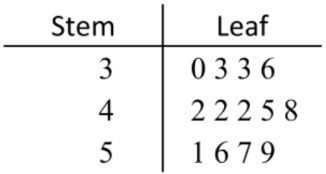# Stem and leaf plots

### Stem and leaf plots

#### Lessons

In this lesson, we will learn:

• How to read a Stem and Leaf Plot
• How to display a data set in a Stem and Leaf Plot
• Finding the mean, median, mode and range for a stem and leaf plot

Notes:

• A Stem and Leaf Plot is a good way to represent data. The data is split into a "stem" which represents the first digit, and a "leaf" which represents the last digit.
• Another way to understand these parts are that:
• The stem contains all digits in the tens place and up
• The leaf is the digit in the ones place only
• Introduction
What are Stem and Leaf Plots?

• 1.
Interpreting a Stem and Leaf Plot
A Stem and Leaf Plot representing the ages of people being immunized against a certain infection.
 Stem: Leaf: 0 145579 1 345569 2 001358

Write out a list of all the numerical data

• 2.
Sandra's class brought all their animals into school one day. The weight of each animal was measured and then displayed in the following Stem and Leaf Plot:
 Weight of Animal in Pounds Stem: Leaf: 0 1146778 1 0135 2 258
a)
What was the combined weight of every single animal that was taken into school that day?

b)
What was the range of animal weights?

• 3.
Creating a Stem and Leaf Plot
A 100m swimming race took place and the time it took the competing swimmers to finish was recorded:
 8.7 seconds 8.5 seconds 9.3 seconds 10.2 seconds 9.5 seconds 9.8 seconds 10.0 seconds 8.1 seconds 8.7 seconds 9.3 seconds 9.0 seconds 10.5 seconds 9.8 seconds 10.2 seconds 8.9 seconds 9.4 seconds
a)
Create a stem and leaf plot

b)
Sometimes when we have too much data lumped into a category we like to split each stem into two or more categories. This sometimes makes the data more nicely represented. For the above question split each stem into two equal parts.

• 4.
Back-to-back Stem and Leaf Plot
It is often useful to consider two data sets to each other. A "Back-to-Back Stem and Leaf Plot" is a good way of handily comparing two distributions.
Meghan's basketball team has played two seasons so far. The amount of points her team scored in each game is given in the following back-to-back stem and leaf plot:
 Amount of Points Scored 1st Season 2nd Season 467 7 88 017 8 369 38 9 47 05 10 1478

List the amount of points her team scored for all their games for each season.

• 5.
Recognizing the leaf and stem of numbers
a)
Underline the leaf of the number
1. 12
2. 30
3. 456

b)
Circle the stem of the number
1. 7
2. 89
3. 231

c)
Write a number with:
1. a stem of 0
2. a leaf of 5
3. a stem of 123.

d)
Circle the pairs of numbers with the same stem
1. 61, 64, 74
2. 23, 230, 235
3. 1890, 189, 1899

e)
Which number would have the same number of digits?
1. Two numbers with the same stem
2. Two numbers with the same leaf

• 6.
Ordering numbers using a stem and leaf plot
Sort the data list using a stem and leaf plot

26, 8, 4, 15, 7, 11, 19, 28, 23, 16a)
What are the stems in the data set? Write them in order from least to greatest in the stem column of the plot.

b)
Write the leaves for the numbers into the stem and leaf plot.

c)
Order the leaves (digits) in each row from least to greatest

d)
Use the stem and leaf plot you have completed to list all the data values from least to greatest.

• 7.
Finding the mean, median, mode and range for a stem and leaf plota)
What is the range for the data in this stem and leaf plot?

b)
What is the median for the data in this stem and leaf plot?

c)
What is the mode for the data in this stem and leaf plot?

d)
What is the mean for the data in this stem and leaf plot? Give the answer with 2 decimal places.